## General Model

This workflow introduces some of the microbial explicit models available in SoilR that include nonlinear interactions. Models of soil organic matter decomposition can be generalized by the equation (Sierra and Müller 2015)

$\frac{d{\bf C}}{dt} = {\bf I}(t) + {\bf N}({\bf C}, t) \cdot {\bf T}({\bf C}, t) \cdot {\bf C}(t)$

which accounts for nonlinear interactions among different pools. This model is implemented in SoilR by the function GeneralNlModel. The functions TwopMMmodel, ThreepairMMmodel, bacwaveModel, and AWBmodel, are specific implementations of microbial nonlinear models that will be described in detail below.

## Two-pool Michaelis-Menten Model: TwopMMmodel

As an example on how the general model can represent an existing model as special case, consider a nonlinear system with two pools: a carbon substrate $$C_S$$ and microbial biomass $$C_B$$ (Manzoni and Porporato 2007). This system can be represented by the coupled first-order ODEs

$\frac{dC_S}{dt} = I + k_B C_B -k_S C_B \frac{C_S}{K_M +C_S} \notag \\ \frac{dC_B}{dt} = (1-r) k_S C_B \frac{C_S}{K_M +C_S} - k_B C_B \notag$ where $$r$$ is the proportion of the decomposed substrate respired by the microbial biomass, and $$k_S$$, $$k_B$$, and $$K_M$$ are constants. This systems can be represented following the general model as $\frac{d{\bf C}}{dt} = \left( \begin{array}{c} I \\ 0 \end{array} \right) + \left( \begin{array}{cc} -1 & 1 \\ (1-r) & -1 \end{array} \right) \left( \begin{array}{cc} \frac{k_S C_B}{K_M +C_S} & 0 \\ 0 & k_B \end{array} \right) \left( \begin{array}{c} C_S \\ C_B \end{array} \right). \notag$

The implementation of this model in SoilR contains the following default values

TwopMMmodel(t, ks = 1.8e-05, kb = 0.007, Km = 900, r = 0.6, Af = 1, ADD = 3.2, ival)


where ADD is equivalent to $$I$$. For details about untis see the function documentation ?TwopMMmodel.

To run the model, we only need to create a vector of time-steps and give initial values to each of the pools.

library(SoilR)
days=seq(0,1000,0.5)

#Run the model with default parameter values
MMmodel=TwopMMmodel(t=days,ival=c(100,10))
Cpools=getC(MMmodel)

#Time solution
matplot(days,Cpools,type="l",ylab="Concentrations",xlab="Days",lty=1,ylim=c(0,max(Cpools)*1.2))
legend("topleft",c("SOM-C", "Microbial biomass"),lty=1,col=c(1,2),bty="n")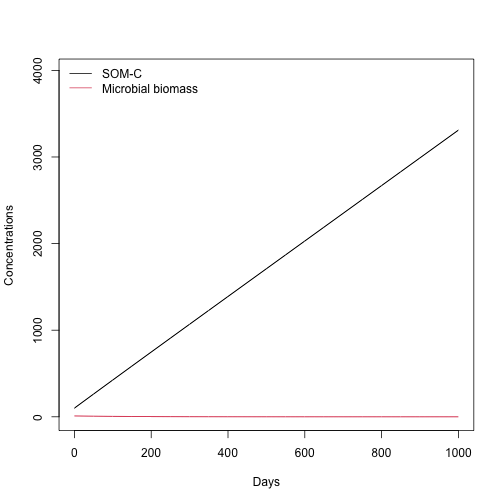## Three-pair Michaelis Menten Model: ThreepMMmodel

One advantage of our implementation of the General Model above is that we can implement models with any number of pools, connections among them, and nonlinear interactions. As an example, consider the following model with three pairs of substrates and microbial biomass

$\frac{d{\bf C}}{dt} = \left( \begin{array}{c} I_1 \\ 0 \\ I_2 \\ 0 \\ I_3 \\ 0 \end{array} \right) + \left( \begin{array}{cccccc} -1 & 1 & 0 & 0 & 0 & 0 \\ (1-r) & -1 & 0 & 0 & 0 & 0 \\ 0 & 0 & -1 & 1 & 0 & 0 \\ 0 & 0 & (1-r) & -1 & 0 & 0 \\ 0 & 0 & 0 & 0 & -1 & 1 \\ 0 & 0 & 0 & 0 & (1-r) & -1 \end{array} \right) \left( \begin{array}{cccccc} \frac{k_{S1} C_{B1}}{K_{M1} +C_{S1}} & 0 & 0 & 0 & 0 & 0 \\ 0 & k_{B1} & 0 & 0 & 0 & 0 & 0 \\ 0 & 0 & \frac{k_{S2} C_{B2}}{K_{M2} +C_{S2}} & 0 & 0 & 0 & 0 \\ 0 & 0 & 0 & k_{B2} & 0 & 0 \\ 0 & 0 & 0 & 0 & \frac{k_{S3} C_{B3}}{K_{M3} +C_{S3}} & 0 \\ 0 & 0 & 0 & 0 & 0 & k_{B3} \end{array} \right) \left( \begin{array}{c} C_{S1} \\ C_{B1} \\ C_{S2} \\ C_{B2} \\ C_{S3} \\ C_{B3} \end{array} \right), \notag$

which is implemented with the function

ThreepairMMmodel(t, ks, kb, Km, r, Af = 1, ADD, ival)


Using arbitrary parameter values and initial conditions, we can run this model and obtain

days=seq(0,1000)
MMmodel=ThreepairMMmodel(t=days,ival=rep(c(100,10),3),ks=c(0.1,0.05,0.01),
kb=c(0.005,0.001,0.0005),Km=c(100,150,200),r=c(0.9,0.9,0.9),
Cpools=getC(MMmodel)

#Time solution
matplot(days,Cpools,type="l",ylab="Concentrations",xlab="Days",lty=rep(1:2,3),
ylim=c(0,max(Cpools)*1.2),col=rep(1:3,each=2),main="Multi-substrate microbial model")
legend("topright",c("Substrate 1", "Microbial biomass 1",
"Substrate 2", "Microbial biomass 2",
"Substrate 3", "Microbial biomass 3"),lty=rep(1:2,3),col=rep(1:3,each=2),
bty="n")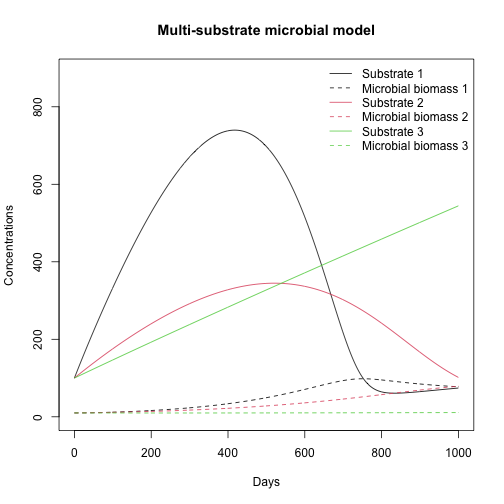#State-space diagram
plot(Cpools[,2],Cpools[,1],type="l",ylab="Substrate",xlab="Microbial biomass")
lines(Cpools[,4],Cpools[,3],col=2)
lines(Cpools[,6],Cpools[,5],col=3)
legend("topright",c("Substrate-Enzyme pair 1","Substrate-Enzyme pair 2",
"Substrate-Enzyme pair 3"),col=1:3,lty=1,bty="n")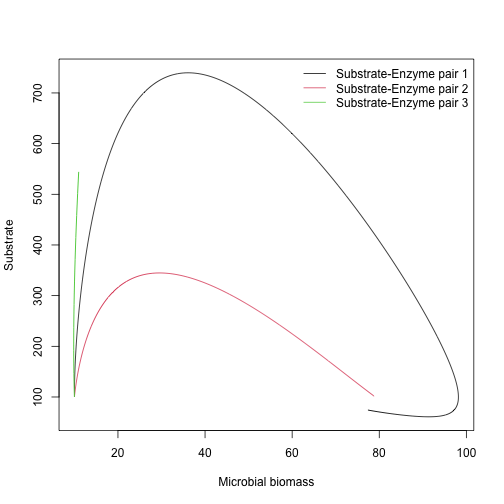## Other models: bacwaveModel and AWBmodel

We also implemented other models of interest, such as the Bacwave model of Zelenev et al. (2000) or the model of Allison et al. (2010).

An example with the Bacwave model with default parameter values from the original publication yields:

hours=seq(0,800,0.1)

#Run the model with default parameter values
bcmodel=bacwaveModel(t=hours)
Cpools=getC(bcmodel)

#Time solution
matplot(hours,Cpools,type="l",ylab="Concentrations",xlab="Hours",lty=1,ylim=c(0,max(Cpools)*1.2))
legend("topleft",c("Substrate", "Microbial biomass"),lty=1,col=c(1,2),bty="n")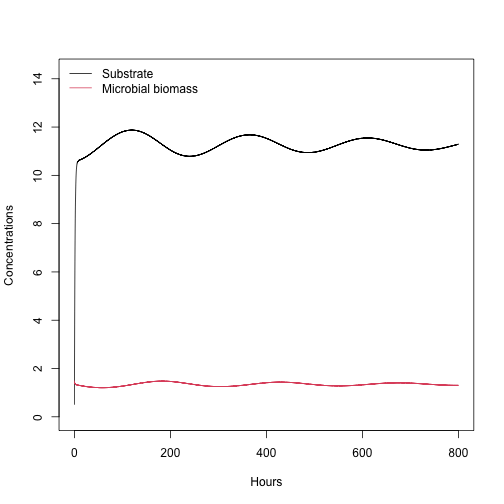#State-space diagram
plot(Cpools[,2],Cpools[,1],type="l",ylab="Substrate",xlab="Microbial biomass")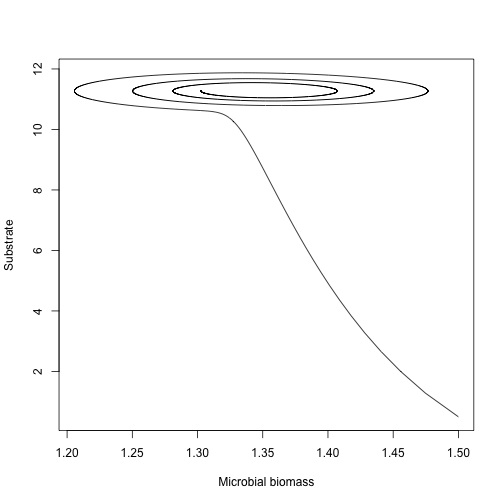The model of Allison, Wallenstein and Bradford (2010) AWBmodel yields:

hours=seq(0,800,0.1)

#Run the model with default parameter values
AWB=AWBmodel(t=hours)
Cpools=getC(AWB)

#Time solution
matplot(hours,Cpools,type="l",ylab="Concentrations",xlab="Hours",lty=1,ylim=c(0,max(Cpools)*1.2))
legend("topleft",c("B", "E", "S", "D"),lty=1,col=c(1:4),bty="n")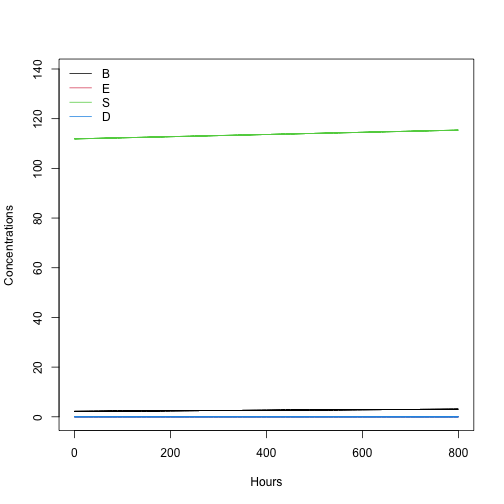## Implement your own nonlinear model

The main function that implements nonlinear models in SoilR is GeneralNlModel. To implement your own nonlinear model, check out the implementation of the models above by typing the function name in the R console.

## References

Allison, S.D., M.D. Wallenstein, M.A. Bradford. 2010. Soil-carbon response to warming dependent on microbial physiology. Nature Geoscience 3: 336-340.

Manzoni, S, A. Porporato (2007). A theoretical analysis of nonlinearities and feedbacks in soil carbon and nitrogen cycles. Soil Biology and Biochemistry 39: 1542-1556.

Sierra, C. A., Müller, M. (2015). A general mathematical framework for representing soil organic matter dynamics. Ecological Monographs, 85, 505–524.

Zelenev, V.V., A.H.C. van Bruggen, A.M. Semenov. 2000. “BACWAVE,” a spatial-temporal model for traveling waves of bacterial populations in response to a moving carbon source in soil. Microbail Ecology 40: 260-2